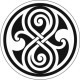# LOG#103. Numbers: the list.Hello, eager earthlings! Today, my list is about fascinating number types. I love them all so much…

1) Natural numbers.

2) Integer numbers.

3) Fractional numbers.

4) Irrational numbers.

5) Real numbers.

6) Complex numbers.

7) Quaternions.

8) Octonions/Octaves/Cayley numbers. (John C. Baez’s favourite numbers!)

9) Sedenions.

10) Hypernumbers (Cayley-Dickson algebras).

11) Grassmann numbers/Grassmannian numbers/anticommuting c-numbers/supernumbers.

12) Clifford numbers.

15) Ternary numbers and n-ary numbers.

16) q-numbers, or xy-qxy, and their q-deformed generalizations.

17) Tropical numbers.

18) Polygonal numbers.

19) Modular numbers.

20) Surreal numbers.

21) Transfinite numbers.

Do you know a cool type of number I should add to “my list”? Let me know…

May the numbers be with you!!!!!

### 2 Comments on “LOG#103. Numbers: the list.”

1.Elangel Exterminador says:

You are definitely on a Power Trip man!

•amarashiki says:

Power trip? Why do you say that? I don’t understand the joke…